# Homology (mathematics)

In mathematics (especially algebraic topology and abstract algebra), homology (in part from Greek ὁμός homos "identical") is a general way of associating a sequence of algebraic objects such as abelian groups or modules to other mathematical objects such as topological spaces. Homology groups were originally defined in algebraic topology. However, similar constructions are available in a wide variety of other contexts, such as groups, Lie algebras, Galois theory, and algebraic geometry.

The original motivation for defining homology groups was the observation that two shapes can be distinguished by examining their holes. For instance, a circle is not a disk because the circle has a hole through it while the disk is solid, and the ordinary sphere is not a circle because the sphere encloses a two-dimensional hole while the circle encloses a one-dimensional hole. However, because a hole is "not there", it is not immediately obvious how to define a hole or how to distinguish different kinds of holes. Homology was originally a rigorous mathematical method for defining and categorizing holes in a manifold. Loosely speaking, a cycle is a closed submanifold, a boundary is the boundary of a submanifold with boundary, and a homology class (which represents a hole) is an equivalence class of cycles modulo boundaries.

There are many different homology theories. A particular type of mathematical object, such as a topological space or a group, may have one or more associated homology theories. When the underlying object has a geometric interpretation like topological spaces do, the nth homology group represents behavior unique to dimension n. In general, most homology groups or modules arise as derived functors on appropriate abelian categories. They provide concrete descriptions of the failure of a functor to be exact. From this abstract perspective, homology groups are determined by objects of a derived category.

## Background

### Origins

Homology theory can be said to start with the Euler polyhedron formula, or Euler characteristic. This was followed by Riemann's definition of genus and n-fold connectedness numerical invariants in 1857 and Betti's proof in 1871 of the independence of "homology numbers" from the choice of basis.

Homology itself was developed as a way to analyse and classify manifolds according to their cycles – closed loops (or more generally submanifolds) that can be drawn on a given n dimensional manifold but not continuously deformed into each other. These cycles are also sometimes thought of as cuts which can be glued back together, or as zippers which can be fastened and unfastened. Cycles are classified by dimension. For example, a line drawn on a surface represents a 1-cycle, a closed loop or(1-manifold), while a surface cut through a three-dimensional manifold is a 2-cycle.

### Surfaces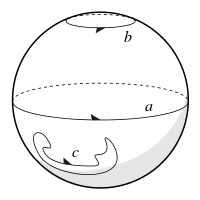Cycles on a 2-sphere

On the ordinary sphere, the cycle b in the diagram can be shrunk to the pole and even the equatorial great circle a can be shrunk in the same way. The Jordan curve theorem shows that any arbitrary cycle such as c can be similarly shrunk to a point. All cycles on the sphere can therefore be continuously transformed into each other and belong to the same homology class. They are said to be homologous to zero. Cutting a manifold along a cycle homologous to zero separates the manifold into two or more components. For example, cutting the sphere along a produces two hemispheres.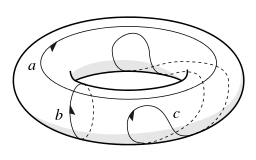Cycles on a torus

This is not generally true of cycles on other surfaces. The torushas cycles which cannot be continuously deformed into each other, for example in the diagram none of the cycles a, b or c can be. Cycles a and b cannot be shrunk significantly. Cycle c can be shrunk to a point, so it is homologous to zero.

If the torus surface is cut along both a and b, it can be opened out and flattened into a rectangle or, more conveniently, a square. One opposite pair of sides represents the cut along a, and the other opposite pair represents the cut along b.

The edges of the square may then be glued back together in different ways. The square can be twisted to allow edges to meet in the opposite direction, as shown by the arrows in the diagram. Up to symmetry, there are four distinct ways of gluing the sides, each creating a different surface: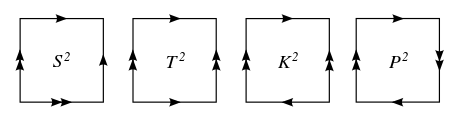The four ways of gluing a square to make a closed surface: glue single arrows together and glue double arrows together.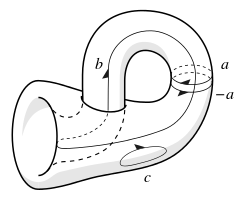Cycles on a Klein bottleis the Klein bottle, which is a torus with a twist in it (The twist can be seen in the square diagram as the reversal of the bottom arrow). It is a theorem that the re-glued surface must self-intersect (when immersed in Euclidean 3-space). Like the torus, cycles a and b cannot be shrunk while c can be. But unlike the torus, following b forwards right round and back reverses left and right, because b happens to cross over the twist given to one join. If an equidistant cut on one side of b is made, it returns on the other side and goes round the surface a second time before returning to its starting point, cutting out a twisted Möbius strip. Because local left and right can be arbitrarily re-oriented in this way, the surface as a whole is said to be non-orientable.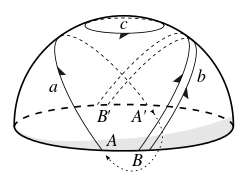Cycles on a hemispherical projective plane

The projective planehas both joins twisted. The uncut form, generally represented as the Boy surface, is visually complex, so a hemispherical embedding is shown in the diagram, in which antipodal points around the rim such as A and A are identified as the same point. Again, a and b are non-shrinkable while c is. But this time, both a and b reverse left and right.

Cycles can be joined or added together, as a and b on the torus were when it was cut open and flattened down. In the Klein bottle diagram, a goes round one way and a goes round the opposite way. If a is thought of as a cut, then −a can be thought of as a gluing operation. Making a cut and then re-gluing it does not change the surface, so a + (a) = 0.

Following an orientable cycle such as a around twice gives simply a + a = 2a. But non-orientable cycles behave differently. In the projective plane, following the unshrinkable cycle b round twice remarkably creates a trivial cycle which can be shrunk to a point; that is, b + b = 0. Because b must be followed around twice to achieve a zero cycle, the surface is said to have a torsion coefficient of 2. The same applies to b in the Klein bottle example.

A square is a contractible topological space, which implies that it has trivial homology. Consequently, additional cuts disconnect it. The square is not the only shape in the plane that can be glued into a surface. Gluing opposite sides of an octagon, for example, produces a surface with two holes. In fact, all closed surfaces can be produced by gluing the sides of some polygon and all even-sided polygons (2n-gons) can be glued to make different manifolds. Conversely, a closed surface with n non-zero classes can be cut into a 2n-gon. Variations are also possible, for example a hexagon may also be glued to form a torus.

The first recognisable theory of homology was published by Henri Poincaré in his seminal paper "Analysis situs", J. Ecole polytech. (2) 1. 1–121 (1895). The paper introduced homology classes and relations. The possible configurations of orientable cycles are classified by the Betti numbers of the manifold (Betti numbers are a refinement of the Euler characteristic). Classifying the non-orientable cycles requires additional information about torsion coefficients.

The complete classification of 1- and 2-manifolds is given in the table.

Topological characteristics of closed 1- and 2-manifolds
Manifold Euler No.
χ
Orientability Betti numbers Torsion coefficient
(1-dimensional)
Symbol Name b0 b1 b2Circle (1-manifold)   0 Orientable 1 1 N/A N/ASphere   2 Orientable 1 0 1 noneTorus   0 Orientable 1 2 1 noneProjective plane   1 Non-orientable 1 0 0 2Klein bottle   0 Non-orientable 1 1 0 2
2-holed torus −2 Orientable 1 4 1 none
g-holed torus (Genus = g) 2 − 2g Orientable 1 2g 1 none
Sphere with c cross-caps 2 − c Non-orientable 1 c − 1 0 2
2-Manifold with g holes
and c cross-caps (c > 0)
2  (2g + c) Non-orientable 1 (2g + c)  1 0 2
NOTES:
1. For a non-orientable surface, a hole is equivalent to two cross-caps.
2. Any 2-manifold is the connected sum of g tori and c projective planes. For the sphere, g = c = 0.

### Generalization

A manifold with boundary or open manifold is topologically distinct from a closed manifold and can be created by making a cut in any suitable closed manifold. For example, the disk or 1-ballis bounded by a circle. It may be created by cutting a trivial cycle in any 2-manifold and keeping the piece removed, by piercing the sphere and stretching the puncture wide, or by cutting the projective plane. It can also be seen as filling-in the circle in the plane.

When two cycles can be continuously deformed into each other, then cutting along one produces the same shape as cutting along the other, up to some bending and stretching. In this case the two cycles are said to be homologous or to lie in the same homology class. Additionally, if one cycle can be continuously deformed into a combination of other cycles, then cutting along the initial cycle is the same as cutting along the combination of other cycles. For example, cutting along a figure 8 is equivalent to cutting along its two lobes. In this case, the figure 8 is said to be homologous to the sum of its lobes.

Two open manifolds with similar boundaries (up to some bending and stretching) may be glued together to form a new manifold which is their connected sum.

This geometric analysis of manifolds is not rigorous. In a search for increased rigour, Poincaré went on to develop the simplicial homology of a triangulated manifold and to create what is now called a chain complex. These chain complexes (since greatly generalized) form the basis for most modern treatments of homology.

In such treatments a cycle need not be continuous: a 0-cycle is a set of points, and cutting along this cycle corresponds to puncturing the manifold. A 1-cycle corresponds to a set of closed loops (an image of the 1-manifold). On a surface, cutting along a 1-cycle yields either disconnected pieces or a simpler shape. A 2-cycle corresponds to a collection of embedded surfaces such as a sphere or a torus, and so on.

Emmy Noether and, independently, Leopold Vietoris and Walther Mayer further developed the theory of algebraic homology groups in the period 1925–28. The new combinatorial topology formally treated topological classes as abelian groups. Homology groups are finitely generated abelian groups, and homology classes are elements of these groups. The Betti numbers of the manifold are the rank of the free part of the homology group, and the non-orientable cycles are described by the torsion part. The subsequent spread of homology groups brought a change of terminology and viewpoint from "combinatorial topology" to "algebraic topology". Algebraic homology remains the primary method of classifying manifolds.

## Informal examples

Informally, the homology of a topological space X is a set of topological invariants of X represented by its homology groupswhere thehomology groupdescribes the k-dimensional holes in X. A 0-dimensional hole is simply a gap between two components, consequentlydescribes the path-connected components of X.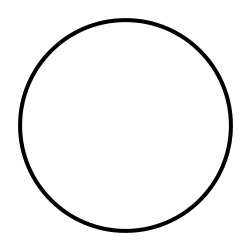The circle or 1-sphereA one-dimensional sphereis a circle. It has a single connected component and a one-dimensional hole, but no higher-dimensional holes. The corresponding homology groups are given aswhereis the group of integers andis the trivial group. The grouprepresents a finitely-generated abelian group, with a single generator representing the one-dimensional hole contained in a circle.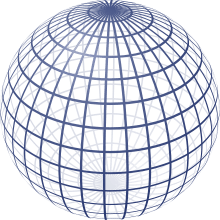The 2-sphereis the shell, not the interior, of a ball

A two-dimensional spherehas a single connected component, no one-dimensional holes, a two-dimensional hole, and no higher-dimensional holes. The corresponding homology groups areIn general for an n-dimensional sphere Sn, the homology groups are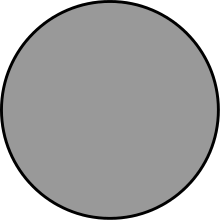The solid disc or 1-ballA one-dimensional ball B1 is a solid disc. It has a single path-connected component, but in contrast to the circle, has no one-dimensional or higher-dimensional holes. The corresponding homology groups are all trivial except for. In general, for an n-dimensional ball Bn,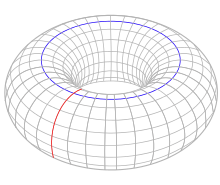The torusThe torus is defined as a Cartesian product of two circles. The torus has a single path-connected component, two independent one-dimensional holes (indicated by circles in red and blue) and one two-dimensional hole as the interior of the torus. The corresponding homology groups areThe two independent 1D holes form independent generators in a finitely-generated abelian group, expressed as the Cartesian product group.

## Construction of homology groups

The construction begins with an object such as a topological space X, on which one first defines a chain complex C(X) encoding information about X. A chain complex is a sequence of abelian groups or modules C0, C1, C2, ... connected by homomorphismswhich are called boundary operators. That is,where 0 denotes the trivial group andfor i < 0. It is also required that the composition of any two consecutive boundary operators be trivial. That is, for all n,i.e., the constant map sending every element of Cn+1 to the group identity in Cn−1. That the boundary of a boundary is trivial implies, wheredenotes the image of the boundary operator andits kernel. Elements ofare called boundaries and elements ofare called cycles.

Since each chain group Cn is abelian all its subgroups are normal. Then becauseandare both subgroups of Cn,is a normal subgroup of. Then one can create the quotient groupcalled the nth homology group of X. The elements of Hn(X) are called homology classes. Each homology class is an equivalence class over cycles and two cycles in the same homology class are said to be homologous.

A chain complex is said to be exact if the image of the (n+1)th map is always equal to the kernel of the nth map. The homology groups of X therefore measure "how far" the chain complex associated to X is from being exact.

The reduced homology groups of a chain complex C(X) are defined as homologies of the augmented chain complexwhere the boundary operatorisfor a combination ∑ niσi of points σi, which are the fixed generators of C0. The reduced homology groupscoincide withfor i ≠ 0. The extrain the chain complex represents the unique mapfrom the empty simplex to X.

Computing the cycleand boundarygroups is usually rather difficult since they have a very large number of generators. On the other hand, there are tools which make the task easier.

The simplicial homology groups Hn(X) of a simplicial complex X are defined using the simplicial chain complex C(X), with Cn(X) the free abelian group generated by the n-simplices of X. The singular homology groups Hn(X) are defined for any topological space X, and agree with the simplicial homology groups for a simplicial complex.

Cohomology groups are formally similar to homology groups: one starts with a cochain complex, which is the same as a chain complex but whose arrows, now denoted dn, point in the direction of increasing n rather than decreasing n; then the groupsof cocycles andof coboundaries follow from the same description. The nth cohomology group of X is then the quotient groupin analogy with the nth homology group.

## Types of homology

The different types of homology theory arise from functors mapping from various categories of mathematical objects to the category of chain complexes. In each case the composition of the functor from objects to chain complexes and the functor from chain complexes to homology groups defines the overall homology functor for the theory.

### Simplicial homology

Main article: Simplicial homology

The motivating example comes from algebraic topology: the simplicial homology of a simplicial complex X. Here An is the free abelian group or module whose generators are the n-dimensional oriented simplexes of X. The mappings are called the boundary mappings and send the simplex with verticesto the sum(which is considered 0 if n = 0).

If we take the modules to be over a field, then the dimension of the n-th homology of X turns out to be the number of "holes" in X at dimension n. It may be computed by putting matrix representations of these boundary mappings in Smith normal form.

### Singular homology

Main article: Singular homology

Using simplicial homology example as a model, one can define a singular homology for any topological space X. A chain complex for X is defined by taking An to be the free abelian group (or free module) whose generators are all continuous maps from n-dimensional simplices into X. The homomorphisms ∂n arise from the boundary maps of simplices.

### Group homology

Main article: Group cohomology

In abstract algebra, one uses homology to define derived functors, for example the Tor functors. Here one starts with some covariant additive functor F and some module X. The chain complex for X is defined as follows: first find a free module F1 and a surjective homomorphism p1 : F1X. Then one finds a free module F2 and a surjective homomorphism p2 : F2 → ker(p1). Continuing in this fashion, a sequence of free modules Fn and homomorphisms pn can be defined. By applying the functor F to this sequence, one obtains a chain complex; the homology Hn of this complex depends only on F and X and is, by definition, the n-th derived functor of F, applied to X.

## Homology functors

Chain complexes form a category: A morphism from the chain complex (dn: AnAn-1) to the chain complex (en: BnBn-1) is a sequence of homomorphisms fn: AnBn such thatfor all n. The n-th homology Hn can be viewed as a covariant functor from the category of chain complexes to the category of abelian groups (or modules).

If the chain complex depends on the object X in a covariant manner (meaning that any morphism X → Y induces a morphism from the chain complex of X to the chain complex of Y), then the Hn are covariant functors from the category that X belongs to into the category of abelian groups (or modules).

The only difference between homology and cohomology is that in cohomology the chain complexes depend in a contravariant manner on X, and that therefore the homology groups (which are called cohomology groups in this context and denoted by Hn) form contravariant functors from the category that X belongs to into the category of abelian groups or modules.

## Properties

If (dn: AnAn-1) is a chain complex such that all but finitely many An are zero, and the others are finitely generated abelian groups (or finite-dimensional vector spaces), then we can define the Euler characteristic(using the rank in the case of abelian groups and the Hamel dimension in the case of vector spaces). It turns out that the Euler characteristic can also be computed on the level of homology:and, especially in algebraic topology, this provides two ways to compute the important invariant χ for the object X which gave rise to the chain complex.

Every short exact sequenceof chain complexes gives rise to a long exact sequence of homology groupsAll maps in this long exact sequence are induced by the maps between the chain complexes, except for the maps Hn(C)Hn-1(A) The latter are called connecting homomorphisms and are provided by the zig-zag lemma. This lemma can be applied to homology in numerous ways that aid in calculating homology groups, such as the theories of relative homology and Mayer-Vietoris sequences.

## Applications

### Application in pure mathematics

Notable theorems proved using homology include the following:

### Application in science and engineering

In topological data analysis, data sets are regarded as a point cloud sampling of a manifold or algebraic variety embedded in Euclidean space. By linking nearest neighbor points in the cloud into a triangulation, a simplicial approximation of the manifold is created and its simplicial homology may be calculated. Finding techniques to robustly calculate homology using various triangulation strategies over multiple length scales is the topic of persistent homology.

In sensor networks, sensors may communicate information via an ad-hoc network that dynamically changes in time. To understand the global context of this set of local measurements and communication paths, it is useful to compute the homology of the network topology to evaluate, for instance, holes in coverage.

In dynamical systems theory in physics, Poincaré was one of the first to consider the interplay between the invariant manifold of a dynamical system and its topological invariants. Morse theory relates the dynamics of a gradient flow on a manifold to, for example, its homology. Floer homology extended this to infinite-dimensional manifolds. The KAM theorem established that periodic orbits can follow complex trajectories; in particular, they may form braids that can be investigated using Floer homology.

In one class of finite element methods, boundary-value problems for differential equations involving the Hodge-Laplace operator may need to be solved on topologically nontrivial domains, for example, in electromagnetic simulations. In these simulations, solution is aided by fixing the cohomology class of the solution based on the chosen boundary conditions and the homology of the domain. FEM domains can be triangulated, from which the simplicial homology can be calculated.

## Software

Various software packages have been developed for the purposes of computing homology groups of finite cell complexes. Linbox is a C++ library for performing fast matrix operations, including Smith normal form; it interfaces with both Gap and Maple. Chomp, CAPD::Redhom and Perseus are also written in C++. All three implement pre-processing algorithms based on Simple-homotopy equivalence and discrete Morse theory to perform homology-preserving reductions of the input cell complexes before resorting to matrix algebra. Kenzo is written in Lisp, and in addition to homology it may also be used to generate presentations of homotopy groups of finite simplicial complexes. Gmsh includes a homology solver for finite element meshes, which can generate Cohomology bases directly usable by finite element software.

## Notes

1. Stillwell 1993, p. 170
2. Weibel 1999, pp. 2–3 (in PDF)
3. Richeson 2008 p.254.
4. Weeks, J.R.; The Shape of Space, CRC Press, 2002.
5. Richeson (2008)
6. Richeson 2008 p.258
7. Weibel 1999, p. 4
8. Hilton 1988, p. 284
9. For example L'émergence de la notion de groupe d'homologie, Nicolas Basbois (PDF), in French, note 41, explicitly names Noether as inventing the homology group.
10. Hirzebruch, Friedrich, Emmy Noether and Topology in Teicher 1999, pp. 61–63.
11. Bourbaki and Algebraic Topology by John McCleary (PDF) gives documentation (translated into English from French originals).
12. Richeson 2008 p.264.
13. Spanier 1966, p. 155
14. Gowers 2010, pp. 390–391
15. Hatcher 2002, p. 106
16. Hatcher 2002, p. 106
17. Hatcher 2002, pp. 105–106
18. Hatcher 2002, p. 113
19. Hatcher 2002, p. 110
20. Spanier 1966, p. 156
21. "CompTop overview". Retrieved 16 March 2014.
22. "Robert Ghrist: applied topology". Retrieved 16 March 2014.
23. van den Berg, J.B.; Ghrist, R.; Vandervorst, R.C.; Wójcik, W. (2015). "Braid Floer homology" (PDF). Journal of Differential Equations. 259 (5): 1663–1721. doi:10.1016/j.jde.2015.03.022.
24. Pellikka, M; S. Suuriniemi; L. Kettunen; C. Geuzaine (2013). "Homology and Cohomology Computation in Finite Element Modeling". SIAM J. Sci. Comput. 35 (5): B1195–B1214. doi:10.1137/130906556.
25. Arnold, Douglas N.; Richard S. Falk; Ragnar Winther (16 May 2006). "Finite element exterior calculus, homological techniques, and applications". Acta Numerica. 15: 1–155. doi:10.1017/S0962492906210018.